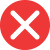61st

Correct spelling, explanation: this is the correct form, as the number ends with ordinal number first, to which we use ending st. Because of this, we write this number as 61st. 61th is incorrect, because th is used for other numbers (with such sound at the end), for example 55th or 784th. Therefore 61st is the only correct form here.

Definition of 61st:
numeral, ordinal number between 60th and 62nd
The library is on 61st street.
Lucy’s grandparents are having their 61st wedding anniversary this week. Amazing!61th

Incorrect spelling, explanation: This form is incorrect because it uses a wrong ending. The correct form is 61st, because th is an ending for ordinal numbers from 4th up, so number 1, 2 and 3 in their ordinal forms have irregular endings (respectively 1st, 2nd and 3rd). Same is done to other ordinal numbers ending with 1st, 2nd and 3rd. Therefore the correct form here is 61st not 61th.61nd

Incorrect spelling61rd

Incorrect spelling